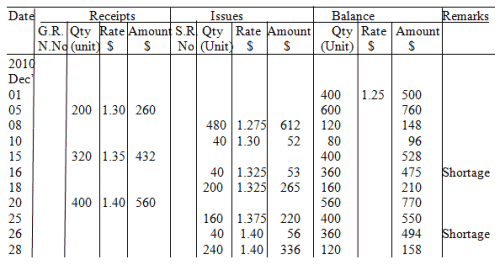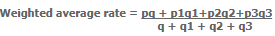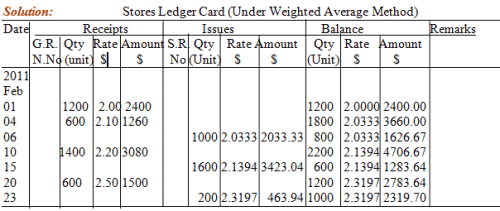Simple and Weighted Averages - Measures of Central Tendency, Business Mathematics & Statistics

# Simple and Weighted Averages - Measures of Central Tendency, Business Mathematics & Statistics - Business Mathematics and Statistics - B Com

## Simple, Weighted Average Methods

1(C)(i) Simple Average Method:

Under this method, simple average rate at cost is obtained by adding the rate of purchases represented by stock at the time of issue & then dividing the same by the number of such rates. The rate needs to be revised at the time of any new purchase or exhaustion of any existing stock. For the purpose of ascertaining the average rate, the quantity by which each purchase is made has to be ignored.
To dampen the severity of the effect of rises & falls in the purchase price, use of any kind of average rate is made. Thus, in case of fluctuating rates of purchase, average cost is used. However, obviously, cost does not get properly represented by the average cost.

(a) It is simple to work out & apply.
(b) Issue price cannot be affected considerably by the fluctuations in prices of purchase.
(c) Average cost method is suitable for the condition when different lots of purchases get mixed up so that the identification is not possible.
(d) Where the quantity of each purchase is stable but the prices fluctuate, average cost method suits the condition.

(a) Profit or loss in material arises as total cost incurred usually does not become equal to the total charges.
(b) Frequent calculations of rates will be necessary in case of frequent purchases, thereby involving much clerical work. Average rate may have to be revised due to exhaustion of an existing stock even if no new purchase comes.
(c) Too much profit or loss on materials may be resulted from the method, when lots of purchases vary much in quantities.
(d) Due to fact that the identity of the materials disappeared in the store, the verification of closing stock figures becomes difficult.
(e) Absurd figures may be shown by the closing stock. The closing stock account may even show credit balance, in times of inflationary spiraling.
The simple average method can work well where:

(a) in each lot, there is standard quantity of purchase
(b) there is very mild fluctuation in prices.

Illustration 4:

From the details prepare stores ledger under simple average method.
2010 Dec 1      Opening Balance         400 kg @ \$ 1.25
5      Received                     200 kg @ \$ 1.30
8      Issued                          480 kg
10    Issued                            40 kg
15    Received                     320 kg @ \$ 1.35
18    Issued                          200 kg
20    Received                     400 kg @ \$ 1.40
25    Issued                          160 kg
28    Issued                          240 kg

Shortage of 40 kg on 16.12.2010 & another shortage of 40 kg on 26.12.2010 is found by the stock verifier.Workings: Calculation of simple average price:-
For Issue on 8th Dec = (1.25+1.30)/2        = \$ 1.275
For Issue on 10th Dec                                 = \$ 1.30
For Shortage on 16th Dec = (1.30+1.35)/2 = \$ 1.325
For Issue on 18th Dec                                  = \$ 1.325
For Issue on 25th Dec = (1.35+1.40)/2        = \$ 1.375
For Shortage on 26th Dec                            = \$ 1.40
For Issue on 28th Dec                                  = \$ 1.40

1(C)(ii) Weighted Average Method:
The weighted average cost under this method is obtained by dividing the total value (at cost) of materials in stock at the time of issue by the total quantity of materials in stock. Only the rates are taken into consideration in case of simple average, on the other hand, the rates & corresponding quantities are considered in case of weighted average because by multiplying the quantity by the rate, the value at cost is obtained. Once a rate is worked out, it goes on being applied until a fresh purchase is made.
If q, q1, q2, & q3 are the quantities in stocks on a day with p, p1, p& p, as the corresponding purchases, the weighted average rate will be worked out as below:(a) The effect of price fluctuations on issue rates are smoothened effectively by the method.
(b) The rate continues in its application unless a new purchase arrives.
(c) Only if, in the calculation of the rates, mathematical approximation is made then profit or loss on materials arises.
(d) Simple & not too much clerical work is involved unless purchases are made frequently.
(e) Where both the price & quantity ordered fluctuate, this method suits the condition.

(a) The work of calculation of rates becomes considerable in case where a frequent purchase is made.
(b) The cost price (nor the market price) of the materials actually issued are not represented by the charges made to issues.
(c) Unless the rates are calculated correcting up to 4 or 5 places of decimal whenever necessary, profit or loss on materials may be created by the method.

Illustration 5:  From the below mentioned details prepare a stores ledger account under weighted average method.

Date                           Particulars                   Units               Unit Cost (\$)
2011Feb         01        Purchases                    1200                           2.00
04        Purchases                      600                           2.10
06        Issues                          1000                             -
10        Purchases                    1400                           2.20
15        Issues                          1600                             -
20        Purchases                      600                           2.50
23        Issues                            200                             -Workings: Calculation of Weighted Average Price-

For Balance of 04th Feb = (2400+1260) / (1200+600)                       = \$ 2.0333

For Balance of 10th Feb = [(2400-2033.33) + 1260+3080]                = \$ 2.1394
[(1200-1000) +600+1400]

For Balance of 20th = (2400+1260+3080+1500-2033.33-3423.04)   = \$ 2.3197
(1200+600+1400+600-1000-1600)

Online Live Tutor Simple Average Method, Weighted Average Method:

We have the best tutors in Economics in the industry. Our tutors can break down a complex Simple Average Method, Weighted Average Method problem into its sub parts and explain to you in detail how each step is performed. This approach of breaking down a problem has been appreciated by majority of our students for learning Simple Average Method, Weighted Average Method concepts. You will get one-to-one personalized attention through our online tutoring which will make learning fun and easy. Our tutors are highly qualified and hold advanced degrees. Please do send us a request for Simple Average Method, Weighted Average Method tutoring and experience the quality yourself.

Online Average Cost Methods Help:
If you are stuck with an Average Cost Methods Homework problem and need help, we have excellent tutors who can provide you with Homework Help. Our tutors who provide Average Cost Methods help are highly qualified. Our tutors have many years of industry experience and have had years of experience providing Average Cost Methods Homework Help. Please do send us the Average Cost Methods problems on which you need help and we will forward then to our tutors for review.

The document Simple and Weighted Averages - Measures of Central Tendency, Business Mathematics & Statistics | Business Mathematics and Statistics - B Com is a part of the B Com Course Business Mathematics and Statistics.
All you need of B Com at this link: B Com

115 videos|142 docs

## FAQs on Simple and Weighted Averages - Measures of Central Tendency, Business Mathematics & Statistics - Business Mathematics and Statistics - B Com

 1. What is a simple average?Ans. A simple average, also known as an arithmetic mean, is a measure of central tendency that is calculated by adding up all the values in a dataset and dividing the sum by the total number of values. It gives us an idea of the typical value in the dataset.
 2. What is a weighted average?Ans. A weighted average takes into account the importance or significance of each value in a dataset. It is calculated by multiplying each value by its corresponding weight, summing up these weighted values, and dividing the sum by the total weight. This type of average is useful when certain values have more influence or impact on the overall average.
 3. How is a simple average calculated?Ans. To calculate a simple average, follow these steps: 1. Add up all the values in the dataset. 2. Count the total number of values. 3. Divide the sum by the total count of values. The result will be the simple average or arithmetic mean.
 4. When should I use a simple average?Ans. A simple average is useful when you want to find the typical value in a dataset without considering any specific importance or weighting of the values. It is commonly used in situations where each value is equally important, or when you want a basic understanding of the central tendency of the data.
 5. In what situations would a weighted average be appropriate?Ans. A weighted average is appropriate when you want to give more importance or significance to certain values in a dataset. This can be useful in situations where the values have different weights or when some values have more impact on the overall average than others. Examples include calculating the weighted average grade in a course, where different assignments or exams carry different weights, or determining the weighted average price of a stock portfolio, where the weight could represent the amount invested in each stock.

115 videos|142 docsExplore Courses for B Com examSignup to see your scores go up within 7 days! Learn & Practice with 1000+ FREE Notes, Videos & Tests.
10M+ students study on EduRev
Track your progress, build streaks, highlight & save important lessons and more!
Related Searches

,

,

,

,

,

,

,

,

,

,

,

,

,

,

,

,

,

,

,

,

,

,

,

,

;